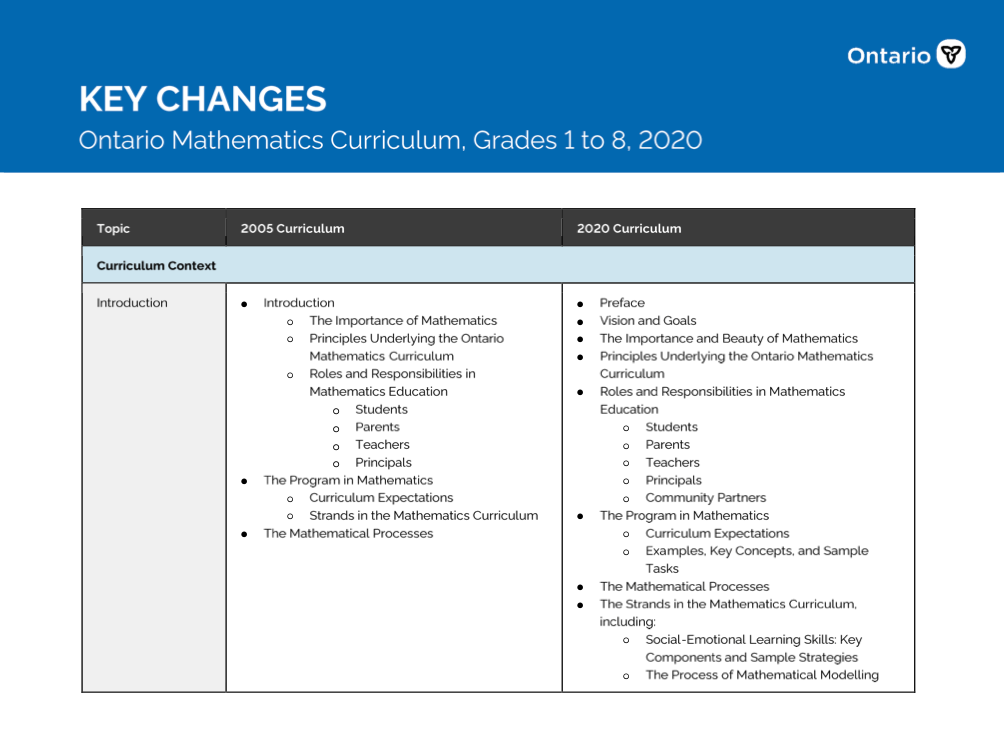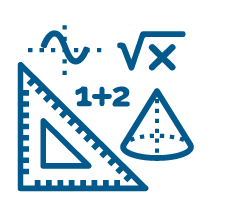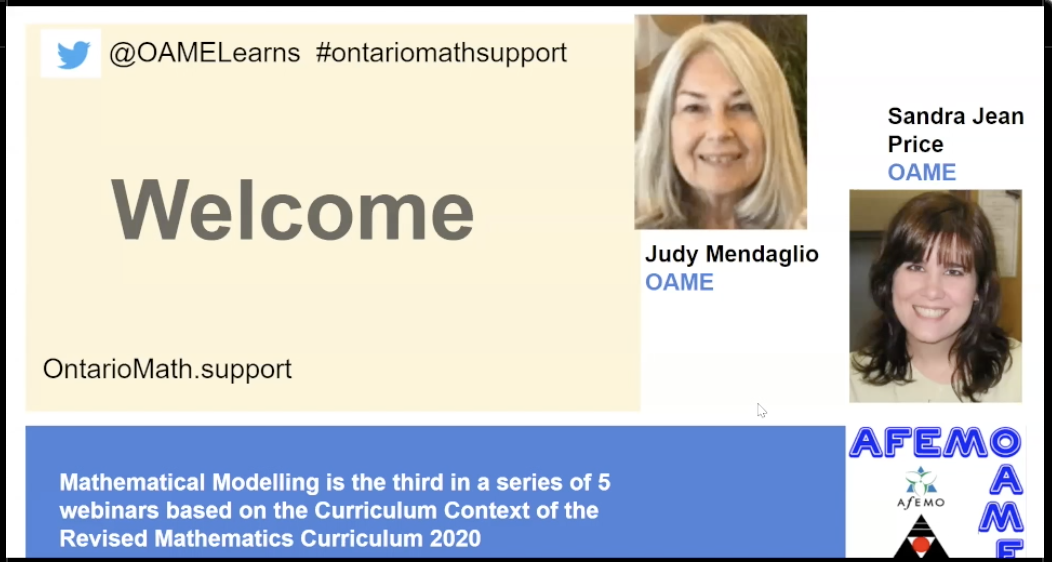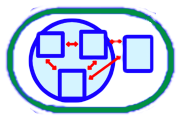# Mathematical Modelling Resources

Coding Number Mathematical Modelling Financial Literacy
All 1 2 3 4 5 6 7 8
Overall Lesson Webinar Recording Webinar Support
Select All    Clear All

NAME
CATEGORYKey Changes - Ontario Mathematics Curriculum, Grades 1 to 8, 2020   [PDF]

This document, prepared by the Ontario Ministry of Education, compares in table form the key changes between the 2005 and the 2020 elementary mathematics curriculum.

OverallOntario Mathematics Curriculum, 2020, Grades 1 - 8   [URL]

This curriculum policy replaces The Ontario Curriculum, Grades 1-8: Mathematics, 2005. Beginning in September 2020, all mathematics programs for Grades 1 to 8 will be based on the expectations outlined in this curriculum policy.

OverallGrade 1 - Mathematical Modelling Lesson - Goody bags for a birthday party   [Folder]

In this lesson, children learn to make a mathematical model in order to predict the number of candies required for a candy bag. Major foci include determining important questions that need answering, missing information that is needed to solve the problem, and making assumptions about missing information.

LessonGrade 1 - Mathematical Modelling Lesson - What should we buy for the dollhouse?   [Folder]

In this lesson, Grade One students develop a mathematical model to try to predict which new dollhouse furniture would most appeal to kindergarten students before it is purchased.

LessonGrade 2 - Mathematical Modelling Lesson - Organizing a Math Manipulatives Area   [Folder]

In this lesson, students will work on the 2 first components of the math modeling process. They will determine how to organize the math manipulatives area for a grade 2 classroom.

LessonGrade 2 - Mathematical Modelling Lesson - Planning an event   [Doc]

In this lesson, students learn to understand the first 2 components of mathematical modeling. They develop a spatial plan for the grade 2 event in the schoolyard.

LessonGrade 2 - Mathematical Modelling Lesson - What inspires you to move during DPA?   [Folder]

Lets create a rating sheet based on criteria to rate how a sequence of movements for DPA can inspire students to move - in this lesson, students learn to understand and develop the 4 components of mathematical modeling. The concept of time is discussed as a possible criteria to rate.

LessonGrade 3 - Mathematical Modelling Lesson - Power Outage   [Folder]

In this lesson students delve into developing questions, determining important information, and considering assumptions within the mathematical modelling process. Using the familiar challenge of adequate power in classroom devices the students work together to ask questions, consider needed information, and determine assumptions so they can articulate a simple model and proceed to collect the data needed. The lesson is followed by lesson two (We Have Power!) where students continue the mathematical modelling process in an iterative fashion.

LessonGrade 3 - Mathematical Modelling Lesson - We have power   [Folder]

In this lesson students continue to develop, analyse, and refine the mathematical model introduced in lesson one (Power Outage!). They have opportunities to collect and interpret additional data to answer additional questions, to create new models, and look for practical solutions to the situation.

LessonGrade 3 - Mathematical Modelling Lesson - Planning a Plant Sale   [Doc]

In this lesson, students have an opportunity to apply mathematical modelling to a real life situation that links directly to their science program. Over a period of several months, they will experience the iterative nature of mathematical modelling as they ask questions, engage in scientific explorations and inquiry, collect data, and develop and analyze their models that will be used for an authentic and valuable purpose in the classroom. The potential culminating task - a plant sale - will also give them chances to apply financial literacy skills as well as engage in numerous writing and media opportunities.

LessonGrade 3 - Mathematical Modelling Lesson - What Size Pots?   [Doc]

In this lesson, students develop mathematical models that consider plant pot sizes and available space using both measurement and number skills. The students engage in all components of the mathematical modelling process in order to solve a classroom issue and determine ways pots can be placed in an organised manner as they grow. In the process, they make direct connections to the science curriculum, and, if desired, the lesson can be linked to math modelling lesson three.

LessonGrade 4 - Mathematical Modelling Lesson - Planning the School Breakfast Club   [Doc]

This lesson is the first of two. Students begin the first three steps of the mathematical modeling process: understanding the situation, analyzing the situation, and creating a mathematical model. From an authentic activity, students work together to come up with a simple model and collect the data. This lesson will be followed by a second lesson called Let's Serve Breakfast! where students continue the mathematical modeling process.

LessonGrade 4 - Mathematical Modelling Lesson - Breakfast is served!   [Doc]

In this lesson, students continue to analyze, evaluate, and modify as necessary their mathematical model presented in Lesson 1 (Planning the School Breakfast Club). They have the opportunity to collect and interpret additional data to answer further questions, create new models, and seek practical solutions to the given situation.

LessonGrade 4 - Mathematical Modelling Lesson - Which in-game purchases are best?   [Folder]

Students will use a mathematical model to help them determine the best in-game purchases to make in a fictional video game scenario.

LessonGrade 5 - Mathematical Modelling Lesson - Choosing DPA Activities   [Folder]

In this lesson, students create a rating scale to help them evaluate and choose DPA activities for their class. They engage in the process of mathematical modelling by working with others to identify assumptions, create a model (their rating system) and then using and revising that model.

LessonGrade 5 - Mathematical Modelling Lesson - Is All Screen Time Created Equally?   [Folder]

In this lesson, students consider the idea of screentime. Many experts think children should limit screentime, and parents want to support their children to start making good choices about amounts of screentime, and what activities might be more or less valuable. Students engage in the process of mathematical modelling to develop a tool that parents and children could use to monitor their screentime, and make good choices about how to spend that time.

LessonGrade 5 - Mathematical Modelling Lesson - Greenhouses in the Arctic   [Folder]

In this lesson, students will consider the question of how fresh produce be reliably supplied to people living in remote Arctic communities. They will consider issues such as transportation costs, the feasibility of building greenhouses, and living in remote communities.

LessonGrade 6 - Mathematical Modelling Lesson - Free Little Library   [Folder]

In this lesson, students find themselves in a messy, real-world problem. A free little library has been donated to the school. “What books are needed to continue to make the little library successful?” Students will make their own choices, assumptions and decisions as they develop a rating system to predict the likelihood a donated book will be read. Suggestions to extend the activity beyond the process of mathematical modelling to what is required to manage a real little library in the school are part of the further consolidation and next steps.

LessonGrade 6 - Mathematical Modelling Lesson - It Pays to Save! Power Up Through Mathematical Modelling   [Folder]

In this 2-part lesson, students will engage in the process of mathematical modelling to produce a model that helps consumers understand their electricity consumption habits and/or which pricing model--Time-of-use or Tiered--is best in meeting their needs.

LessonGrade 6 - Mathematical Modelling Lesson - School Garden   [Folder]

In this lesson, students examine the idea of making a school garden. We live in a vast and varied province. Students develop a model to predict the likelihood of success for a variety of garden plans. The model would be used by others, for example, a parent group, when deciding the winning student-designed garden. Additional activities include information to create a garden and the study of Sustainable Development Goals in Social Studies.

LessonGrade 7 - Mathematical Modelling Lesson - Handwashing Policy   [Folder]

In this lessons students will create a model then collect and organize data in order to determine the impact of a school board's handwashing policy. Students will use their model to make predictions, then test those predictions by playing out the handwashing scenario in real-life. In the end, students will present their opinion on the policy, using mathematical arguements based on information provided by their models.

LessonGrade 7 - Mathematical Modelling Lesson - Forests in Crisis   [Doc]

In this lesson, students will apply the process of mathematical modelling to analyse data about the current rates of deforestation in Canada. Students will create a model that can be used to predict the point at which we will no longer have enough forestry products to meet our needs.

LessonGrade 7 - Mathematical Modelling Lesson - Top 10 Players   [Doc]

In this lesson, students will create a model, then collect and organize data in order to predict the 10 best pro-athletes in a given sport. Students will use their model to make and test predictions about which hockey players should be chosen for a fantasy hockey league.

LessonGrade 8 - Mathematical Modelling Lesson - Let's plan a school trip   [Folder]

In this lesson, students will put their financial literacy and research skills to work as they move through the process of determining the perfect outing for their year-end Grade 8 trip. Students will explore the financial implications, supervision ratios, distance regulations as well as the research the hidden attractions within their community.

LessonGrade 8 - Mathematical Modelling Lesson - Predicting the Next YouTube stars   [Folder]

In this lesson, students will try to discover what it takes to become a Youtube superstar. Students are task with determining the factors required to become one of the most famous youtuber on the planet. Using those factors, students will then try to create a model that will predict and rank the futur stars of the video platform.

LessonGrade 8 - Mathematical Modelling Lesson - How Water Rich is Canada?   [Folder]

In this lesson, math and science meet to form an open and engaging learning opportunity. Students are tasked with examining Canada's water richness to determine at what point will the tables be turned. As students sift through the various factors that contribute to Canada's water richness, supply and use they will begin to reflect on the assumptions they are making to formulate a mathematics model.

LessonWebinar 3 - Mathematical Modelling   [MP4]

This hour-long webinar will focus on Mathematical Modelling. In this webinar, we will explore in detail what the mathematical modelling process looks like and how it is truly new to the curriculum. We hope to deepen educators’ understanding of what Mathematical Modelling is and how it can be woven into a math program. Sample tasks will be discussed.

Webinar RecordingGr 1/2/3 Webinar: Mathematical Modelling in the Primary Grades   [MOV]

Welcome to this webinar about teaching the new Mathematical Modelling expectations for the primary grades of the revised mathematics curriculum. In this webinar, we take you through the lessons that have been created by Ontario educators to support Ontario educators. These Mathematical Modelling lessons have a focus on developing students’ appreciation for the role mathematics plays in real “real life” while providing students with opportunities to experience mathematics in a more personal and relevant way.

Webinar Recording